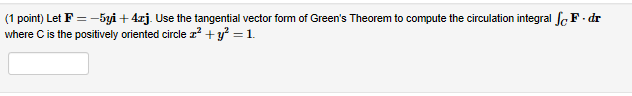# (1 point) Let F--5yi 4zj. Use the tangential vector form of Green's Theorem to compute the circulation integral cF -dr where C is the positively oriented circle z2y1.

Questionhelp_outlineImage Transcriptionclose(1 point) Let F--5yi 4zj. Use the tangential vector form of Green's Theorem to compute the circulation integral cF -dr where C is the positively oriented circle z2y1. fullscreen

### Want to see the step-by-step answer?